﻿ Evaluation of Triple Integrals

Evaluation of Triple Integrals

Chii-Huei Yu, Shinn-Der SheuOPEN ACCESSPEER-REVIEWED

Evaluation of Triple Integrals

Chii-Huei Yu1,, Shinn-Der Sheu2

1Department of Management and Information, Nan Jeon University of Science and Technology, Tainan City, Taiwan

2Department of Information Technology, Nan Jeon University of Science and Technology, Tainan City, Taiwan

Abstract

This article considers two types of triple integrals and uses Maple for verification. The infinite series forms of the two types of triple integrals can be obtained using binomial series and integration term by term theorem. In addition, some examples are used to demonstrate the calculations.

• Yu, Chii-Huei, and Shinn-Der Sheu. "Evaluation of Triple Integrals." American Journal of Systems and Software 2.4 (2014): 85-88.
• Yu, C. , & Sheu, S. (2014). Evaluation of Triple Integrals. American Journal of Systems and Software, 2(4), 85-88.
• Yu, Chii-Huei, and Shinn-Der Sheu. "Evaluation of Triple Integrals." American Journal of Systems and Software 2, no. 4 (2014): 85-88.

 Import into BibTeX Import into EndNote Import into RefMan Import into RefWorks

1. Introduction

In calculus and engineering mathematics, there are many methods to solve the integral problems including change of variables method, integration by parts method, partial fractions method, trigonometric substitution method, etc. In this paper, we study the following two types of triple integrals which are not easy to obtain their answers using the methods mentioned above.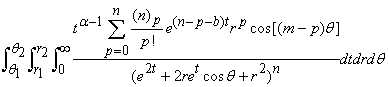(1)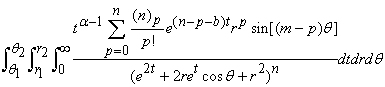(2)

where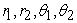are real numbers,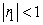,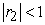,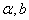are real numbers,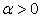,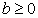, and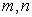are positive integers. We can obtain the infinite series forms of these triple integrals using binomial series and integration term by term theorem; these are the major results of this paper (i.e., Theorems 2.5 and 2.6). Adams et al. , Nyblom , and Oster  provided some techniques to solve the integral problems. Yu [4-29], Yu and B. -H. Chen , Yu and Sheu [31, 32], and T. -J. Chen and Yu [33, 34, 35] used complex power series method, integration term by term theorem, differentiation with respect to a parameter, Parseval’s theorem, area mean value theorem, and generalized Cauchy integral formula to solve some types of integrals. In this paper, two examples are used to demonstrate the proposed calculations, and the manual calculations are verified using Maple.

2. Main Results

Some notations, formulas and theorems used in this paper are introduced below.

2.1. Notations
2.1.1. Gamma Function

Suppose that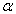is a positive real number, then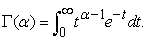2.1.2.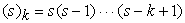, where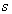is a real number, and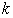is a positive integer;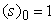.

2.2 Formulas
2.2.1. Euler’s Formula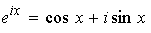, where x is any real number.

2.2.2. DeMoivre’s Formula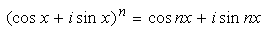, where n is any integer, and x is any real number.

2.3. Theorems

Two important theorems used in this study are introduced below.

2.3.1. Binomial Series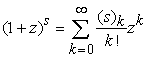, where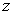is a complex number,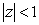, andis a real number.

The following theorem can be found in [, p 269]

2.3.2. Integration Term by Term Theorem

Suppose that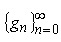is a sequence of Lebesgue integrable functions defined on I. If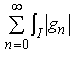is convergent, then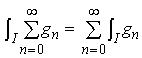.

Before deriving the major results of this study, we need a lemma.

Lemma 2.4. Suppose thatis a complex number,,are real numbers,,, andare positive integers. Then the improper integral: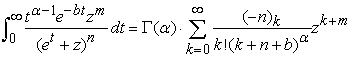(3)

Proof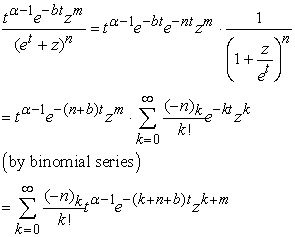(4)

Thus,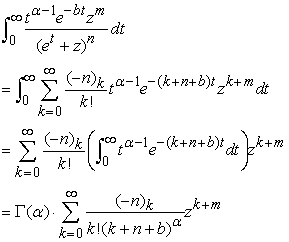Firstly, we determine the infinite series form of the triple integral (1).

Theorem 2.5. Assume thatare real numbers,,,are real numbers,,, andare positive integers. Then the triple integral: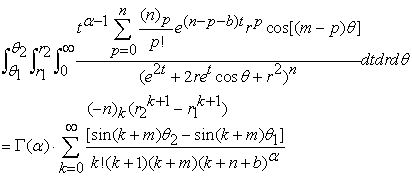(5)

Proof Let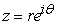in Eq. (3), where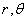are real numbers, and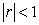, then: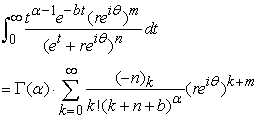(6)

By Euler’s formula and DeMoivre’s formula, we obtain: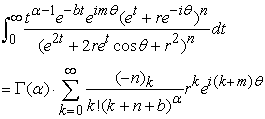(7)

Therefore,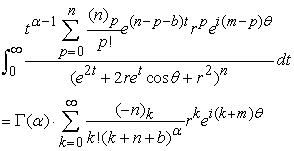(8)

Using the equality of real parts of both sides of Eq. (8) yields: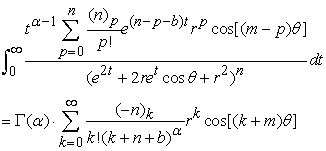(9)

Hence, the triple integral: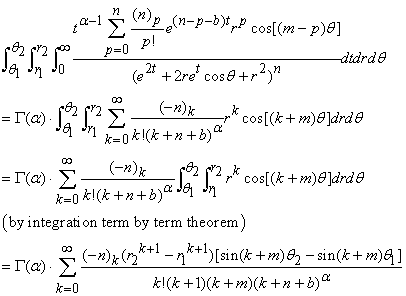Next, we determine the infinite series form of the triple integral (2).

Theorem 2.6. If the assumptions are the same as Theorem 2.5, then the triple integral: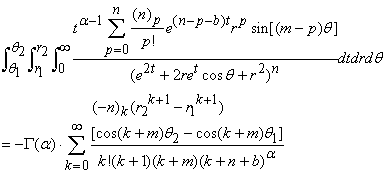(10)

Proof Using the equality of imaginary parts of both sides of Eq. (8) yields: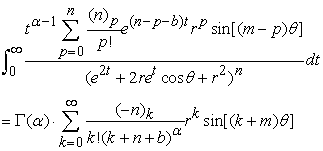(11)

Thus, the triple integral: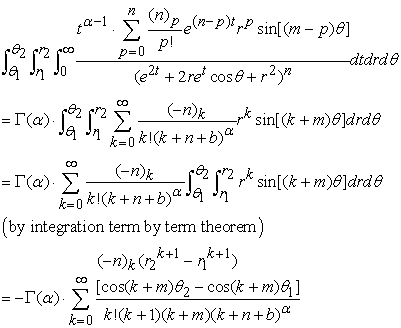3. Examples

In the following, for the two types of triple integrals in this study, we provide two examples and use Theorems 2.5 and 2.6 to find their infinite series forms. On the other hand, Maple is used to calculate the approximations of these triple integrals and their solutions for verifying our answers.

Example 3.1 In Eq. (5), if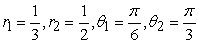,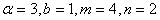, then the triple integral: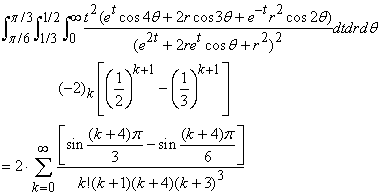(12)

Next, we use Maple to verify the correctness of Eq. (12).

>evalf(Tripleint(t^2*(exp(t)*cos(4*theta)+2*r*cos(3*theta)+exp(-t)*r^2*cos(2*theta))/(exp(2*t)+2*r*exp(t)* cos(theta)+r^2)^2,t=0..infinity,r=1/3..1/2,theta=Pi/6..Pi/3),14);

-0.0042223802575385

>evalf(2*sum(product(-2-j,j=0..(k-1))*((1/2)^(k+1)-(1/3)^(k+1))*(sin((k+4)*Pi/3)-sin((k+4)*Pi/6))/ (k!*(k+1)*(k+4)*(k+3)^3),k=0..infinity),14);

-0.0042223802575385

Example 3.2. In Eq. (10), if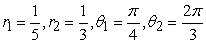,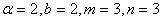, then the triple integral: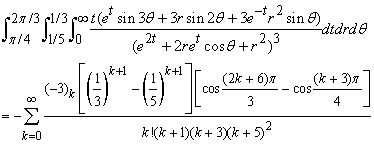(13)

We also use Maple to verify the correctness of Eq. (13).

>evalf(Tripleint(t*(exp(t)*sin(3*theta)+3*r*sin(2*theta)+3*exp(-t)*r^2*sin(theta))/(exp(2*t)+2*r*exp(t)* cos(theta)+r^2)^3,t=0..infinity,r=1/5..1/3,theta=Pi/4..2*Pi/3),14);

-0.0026286382283423

>evalf(-sum(product(-3-j,j=0..(k-1))*((1/3)^(k+1)-(1/5)^(k+1))*(cos((2*k+6)*Pi/3)-cos((k+3)*Pi/4))/ (k!*(k+1)*(k+3)*(k+5)^2),k=0..infinity),14);

-0.0026286282283423

4. Conclusion

In this paper, we use binomial series and integration term by term theorem to solve two types of triple integrals. In fact, the applications of the two methods are extensive, and can be used to easily solve many difficult problems; we endeavor to conduct further studies on related applications. On the other hand, Maple also plays a vital assistive role in problem-solving. In the future, we will extend the research topic to other calculus and engineering mathematics problems and use Maple to verify our answers.

References

  A. A. Adams, H. Gottliebsen, S. A. Linton, and U. Martin, “Automated theorem proving in support of computer algebra: symbolic definite integration as a case study,” Proceedings of the 1999 International Symposium on Symbolic and Algebraic Computation, Canada, pp. 253-260, 1999.In article CrossRef  M. A. Nyblom, “On the evaluation of a definite integral involving nested square root functions,” Rocky Mountain Journal of Mathematics, Vol. 37, No. 4, pp. 1301-1304, 2007.In article CrossRef  C. Oster, “Limit of a definite integral,” SIAM Review, Vol. 33, No. 1, pp. 115-116, 1991.In article CrossRef  C. -H. Yu, “A study of two types of definite integrals with Maple,” Jökull Journal, Vol. 64, No. 2, pp. 543-550, 2014.In article  C. -H. Yu, “Evaluating two types of definite integrals using Parseval’s theorem,” Wulfenia Journal, Vol. 21, No. 2, pp. 24-32, 2014.In article  C. -H. Yu, “Solving some definite integrals using Parseval’s theorem,” American Journal of Numerical Analysis, Vol. 2, No. 2, pp. 60-64, 2014.In article  C. -H. Yu, “Some types of integral problems,” American Journal of Systems and Software, Vol. 2, No. 1, pp. 22-26, 2014.In article  C. -H. Yu, “Using Maple to study the double integral problems,” Applied and Computational Mathematics, Vol. 2, No. 2, pp. 28-31, 2013.In article CrossRef  C. -H. Yu, “A study on double Integrals,” International Journal of Research in Information Technology, Vol. 1, Issue. 8, pp. 24-31, 2013.In article  C. -H. Yu, “Application of Parseval’s theorem on evaluating some definite integrals,” Turkish Journal of Analysis and Number Theory, Vol. 2, No. 1, pp. 1-5, 2014.In article  C. -H. Yu, “Evaluation of two types of integrals using Maple, ”Universal Journal of Applied Science, Vol. 2, No. 2, pp. 39-46, 2014.In article  C. -H. Yu, “Studying three types of integrals with Maple, ”American Journal of Computing Research Repository, Vol. 2, No. 1, pp. 19-21, 2014.In article  C. -H. Yu, “The application of Parseval’s theorem to integral problems,” Applied Mathematics and Physics, Vol. 2, No. 1, pp. 4-9, 2014.In article  C. -H. Yu, “A study of some integral problems using Maple,” Mathematics and Statistics, Vol. 2, No. 1, pp. 1-5, 2014.In article  C. -H. Yu, “Solving some definite integrals by using Maple, ”World Journal of Computer Application and Technology, Vol. 2, No. 3, pp. 61-65, 2014.In article  C. -H. Yu, “Using Maple to study two types of integrals,” International Journal of Research in Computer Applications and Robotics, Vol. 1, Issue. 4, pp. 14-22, 2013.In article  C. -H. Yu, “Solving some integrals with Maple,” International Journal of Research in Aeronautical and Mechanical Engineering, Vol. 1, Issue. 3, pp. 29-35, 2013.In article  C. -H. Yu, “A study on integral problems by using Maple, ”International Journal of Advanced Research in Computer Science and Software Engineering, Vol. 3, Issue. 7, pp. 41-46, 2013.In article  C. -H. Yu, “Evaluating some integrals with Maple,” International Journal of Computer Science and Mobile Computing, Vol. 2, Issue. 7, pp. 66-71, 2013.In article  C. -H. Yu, “Application of Maple on evaluation of definite integrals,” Applied Mechanics and Materials, Vols. 479-480 (2014), pp. 823-827, 2013.In article  C. -H. Yu, “Application of Maple on the integral problems,” Applied Mechanics and Materials, Vols. 479-480 (2014), pp. 849-854, 2013.In article  C. -H. Yu, “Using Maple to study the integrals of trigonometric functions,” Proceedings of the 6th IEEE/International Conference on Advanced Infocomm Technology, Taiwan, No. 00294, 2013.In article  C. -H. Yu, “A study of the integrals of trigonometric functions with Maple,” Proceedings of the Institute of Industrial Engineers Asian Conference 2013, Taiwan, Springer, Vol. 1, pp. 603-610, 2013.In article CrossRef  C. -H. Yu, “Application of Maple on the integral problem of some type of rational functions,” (in Chinese) Proceedings of the Annual Meeting and Academic Conference for Association of IE, Taiwan, D357-D362, 2012.In article  C. -H. Yu, “Application of Maple on some integral problems, ”(in Chinese) Proceedings of the International Conference on Safety & Security Management and Engineering Technology 2012, Taiwan, pp. 290-294, 2012.In article  C. -H. Yu, “Application of Maple on some type of integral problem,” (in Chinese) Proceedings of the Ubiquitous-Home Conference 2012, Taiwan, pp. 206-210, 2012.In article  C. -H. Yu, “Application of Maple on evaluating the closed forms of two types of integrals,” (in Chinese) Proceedings of the 17th Mobile Computing Workshop, Taiwan, ID16, 2012.In article  C. -H. Yu, “Application of Maple: taking two special integral problems as examples,” (in Chinese) Proceedings of the 8th International Conference on Knowledge Community, Taiwan, pp. 803-811, 2012.In article  C. -H. Yu, “Evaluating some types of definite integrals,” American Journal of Software Engineering, Vol. 2, Issue. 1, pp. 13-15, 2014.In article  C. -H. Yu and B. -H. Chen, “Solving some types of integrals using Maple,” Universal Journal of Computational Mathematics, Vol. 2, No. 3, pp. 39-47, 2014.In article  C. -H. Yu and S. -D. Sheu “Infinite series forms of double integrals,” International Journal of Data Envelopment Analysis and *Operations Research*, Vol. 1, No. 2, pp. 16-20, 2014.In article  C. -H. Yu and S. -D. Sheu “Using area mean value theorem to solve some double integrals,” Turkish Journal of Analysis and Number Theory, Vol. 2, No. 3, pp. 75-79, 2014.In article  T. -J. Chen and C. -H. Yu, “A study on the integral problems of trigonometric functions using two methods,” Wulfenia Journal, Vol. 21, No. 4, pp. 76-86, 2014.In article  T. -J. Chen and C. -H. Yu, “Fourier series expansions of some definite integrals,” Sylwan Journal, Vol. 158, Issue. 5, pp. 124-131, 2014.In article  T. -J. Chen and C. -H. Yu, “Evaluating some definite integrals using generalized Cauchy integral formula,” Mitteilungen Klosterneuburg, Vol. 64, Issue. 5, pp. 52-63, 2014.In article  T. M. Apostol, Mathematical analysis (2nd ed.), Addison-Wesley, Massachusetts, 1975.In article